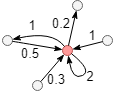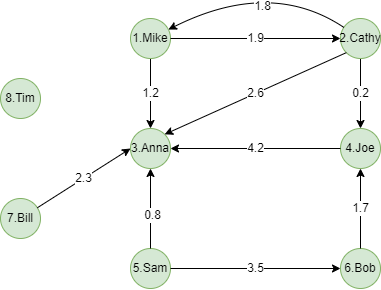# Change Nickname

Current Nickname:
Search
v4.0
v4.0

# Degree

## Overview

The Degree algorithm calculates the number of edges for each node, and the sum of weights when there are weights on the edges. Since it is a shallow (≤ 1 layer) calculation for nodes, degree is the simplest and most efficient graph algorithm, which plays a vital role in scientific computing, feature extraction, supernode recognition and other fields.

## Basic Concept

### Degree Direction

The number of outbound edges of node is called out degree of the node; conversely, the number of inbound edges of node is called in degree of the node. If ignores the direction of edges, that is degree (or degree centrality) of the node.Out degree of the red node in the graph above is `3`, in degree is `4`, degree is `7`. Please note that the directed self-loop edge is regarded as an outbound edge and an inbound edge.

### Edge Weight

Edge weight can be a property value of edge. When edge weights are specified, degree is the sum of all edge weights. The degree of node without specifying edge weights can be viewed as the degree when all edge weights are 1.After the edges are weighted, out degree of the red node in the graph above is `1 + 0.2 + 2 = 3.2`, in degree is `0.5 + 0.3 + 2 + 1 = 3.8`, degree is `3.2 + 3.8 = 7`.

## Special Case

### Lonely Node, Disconnected Graph

Degree of lonely node all depends on its self-loop edge because it has no edge connected to other nodes.

### Self-loop Edge

Self-loop edge is regarded as an outbound edge and an inbound edge.

### Directed Edge

The direction of directed edge is the basis for calculating out degree and in degree; the direction of edge is ignored when calculating degree.

## Results and Statistics

Take the 8-people small social network below as an example, run the Degree algorithm against all nodes:Algorithm results: Calculate degree for each node, return `_id`, `degree` or `_uuid`, `degree` according to the execution method

_uuid _id degree
1 Mike 3
2 Cathy 3
3 Anna 5
4 Joe 2
5 Sam 2
6 Bob 2
7 Bill 1
8 Timmy 0

Algorithm statistics: Total degree `total_degree` and average degree `average_degree` of all nodes

total_degree average_degree
18 2.25

## Command and Configuration

• Command: `algo(degree)`
• Configurations for the parameter `params()`:
Name Type
Default
Specification
Description
ids / uuids []`_id` / []`_uuid` / / IDs or UUIDs of nodes to be calculated; all nodes to be calculated if not set
edge_schema_property []`@<schema>?.<property>` / Numeric edge property, LTE needed Edge weight property/properties, schema can be either carried or not; edge without any specified property does not participate in the calculation; degree is unweighted if not set
direction string / in/out, case insensitive Edge direction; direction ignored if not set
limit int -1 >=-1 Number of results to return; return all results if sets to -1 or not set
order string / ASC/DESC, case insensitive To sort the returned results; no sorting is applied if not set

Example: Calculate out degree of node UUID = 1

``````algo(degree).params({
uuids: ,
direction: "out"
}) as d1
return d1
``````

Example: Calculate degree of nodes ID = Sam, Anna, Mike

``````algo(degree).params({
ids: ["Sam", "Anna" , "Mike"]
}) as degree
return degree
``````

Example: Calculate in degree of all nodes, edges are weighted by properties @follow.score, return the first 5 maximum results

``````algo(degree).params({
direction: "in",
edge_schema_property: @follow.score,
limit: 5
}) as degree
return degree
``````

## Algorithm Execution

#### 1. File Writeback

Configuration Data in Each Row
filename `_id`,`degree`

Example: Calculate degree of all nodes, write the algorithm results back to file named degree_all

``````algo(degree).params().write({
file:{
filename: "degree_all"
}
})
``````

#### 2. Property Writeback

Configuration Writeback Content Type
Data Type
property `degree` Node property `int64` when edge is unweighted, `int64` or `double` when edge is weighted

Example: Calculate degree of all nodes, write the degree back to node property named degree

``````algo(degree).params().write({
db:{
property: "degree"
}
})
``````

#### 3. Statistics Writeback

Name Data Type Description
`total_degree` float Total degree of all nodes
`average_degree` float Average degree of all nodes

Example: Calculate degree of all nodes, write the algorithm statistics back to task information

``````algo(degree).params().write()
``````

### Direct Return

Alias Ordinal
Type
Description
Column Name
0 []perNode Node and its degree `_uuid`, `degree`
1 KV Total and average degree of all nodes `total_degree`, `average_degree`

Example: Calculate degree of all nodes, define algorithm results and statistics as aliases named results and stats, and return the results and statistics

``````algo(degree).params() as results, stats
return results, stats
``````

### Streaming Return

Alias Ordinal
Type
Description
Column Name
0 []perNode Node and its degree `_uuid`, `degree`

Example: Calculate degree of all nodes, define algorithm results as alias named results, return the results ordered by the ascending UUID of nodes

``````algo(degree).params().stream() as results
return results order by results._uuid
``````

### Real-time Statistics

Alias Ordinal
Type
Description
Column Name
0 KV Total and average degree of all nodes `total_degree`, `average_degree`

Example: Calculate degree of all nodes, define algorithm statistics as alias named stats, return the statistics

``````algo(degree).params().stats() as stats
return stats
``````